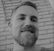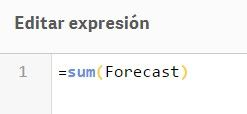Announcements
Applications are open for the 2024 Qlik Luminary Program. Apply by December 15 here.
cancel
Showing results for
Did you mean:Creator

## LOOP not working in Qlik Sense script

Hi,

I am having a problem trying to do a FOR LOOP as it produces no values.

I am doing 3 steps, but not sure what is the problem, I have attached the APP.

1.Determin the FOR values of the variables above.

2. Creating 2 variables

3. Apply the variables to TABLE from a RESIDENT table. But it produces no value after LOADING the script.

``````/// 1. These are the FOR values to PASS for the variables below.

for i= -1 to -7 ;
for j=-8 to -15;
for z= -16 to -21

//// 2.These are variable FUNCTIONS with same structure
LET
V_result1=(sum(Peek(Result_1,\$i))*0.45+sum(Peek(Result_1,\$j))*0.35+sum(Peek(Result_1,\$z))*0.2)*1/5;
V_result2=(sum(Peek(Result_2,\$i))*0.45+sum(Peek(Result_2,\$j))*0.35+sum(Peek(Result_2,\$z))*0.2)*1/5;

//// 3. The table where to apply those VARIABLES from a RESIDENT table.
DATE_PRODUCTION_4;
"Date",
Sum(\$(V_result1)) as Forecast1,
sum(\$(V_result2)) as Forecast2

Resident [DATE_PRODUCTION_3]
GROUP BY "Date";``````

Labels (1)
• ### Looping variable script

1 Solution

Accepted SolutionsPartner - Specialist

I... don't think for loops work like that.

I might be completely off base here, but you seem to be trying to do some kind of moving window calculation, so for each date sum up the previous week and multiply by some weight, the week before previous and multiply by some other weight, and finally the week two weeks before previous and multiply by yet another weight. Finally multiply the total result by yet another factor (1/5). This is done separately for each action, which is why you have multiple result columns.

So assuming you have one row per date, you would want to sum up a total of 21 rows for each date (3*7). With three nested for loops, you'd make a total of 7^3 = 343 iterations for each date. Even if you could use for loop as some kind of generator for the variable formula, which you can't because Qlik script does not do generators.

If my idea of what you are trying to do is correct, I would do it completely differently:

1. Create a date bridge table from master calendar. So, for each date generate "linking dates" going back 3 weeks, using while clause. Additionally create a "week difference" field which would range from 1 to 3
2. Left join your facts (DATE_PRODUCTION_3) to that, using linking dates as key
3. Group the resulting table by date (the original one, not the linking one), with something like Sum(Result * (pick("week difference"), 0.45, 0.35, 0.2)) as the aggregation formula
4. Finally do a resident load from that and multiply each aggregation result by 1/5

7 RepliesMVP

May be this

``````/// 1. These are the FOR values to PASS for the variables below.
for i= -1 to -7
for j=-8 to -15
for z= -16 to -21

//// 2.These are variable FUNCTIONS with same structure
LET V_result1=((Peek(Result_1,\$(i)))*0.45+(Peek(Result_1,\$(j))*0.35+(Peek(Result_1,\$(z)))*0.2)*1/5;
LET V_result2=((Peek(Result_2,\$(i)))*0.45+(Peek(Result_2,\$(j)))*0.35+sum(Peek(Result_2,\$(z)))*0.2)*1/5;

//// 3. The table where to apply those VARIABLES from a RESIDENT table.
DATE_PRODUCTION_4:
Sum(\$(V_result1)) as Forecast1,
sum(\$(V_result2)) as Forecast2
Resident [DATE_PRODUCTION_3]
GROUP BY "Date";

DATE_PRODUCTION_5:
CrossTable ("Accion Marketing",Forecast,1)
Date,
Forecast1,
Forecast2

RESIDENT DATE_PRODUCTION_4;
DROP TABLE DATE_PRODUCTION_4;

NEXT;
NEXT;
NEXT;``````Creator
Author

I have tested but still produce the same result.

Variable FORECAST does not exists.I have TEST the Following:

1) Creat a 3rd variable called Forecast 3 that is not produced froma CALL to variable produce by a LET FOR STATEMENTS.

2) Removing the later step with the crosstable function.

RESULT: Forecast1, Forecast2 and Forecast3 does not exists

Don´t know what I am doing wrong?

`````` DATE_PRODUCTION_4:
Sum(\$(V_result1)) as Forecast1,
sum(\$(V_result2)) as Forecast2,
Peek(Result_2,1)+Peek(Result_2,2) as Forecast3
Resident [DATE_PRODUCTION_3]
GROUP BY "Date";``````Partner - Master III

hi

couple of observations

1. i think a for loop in qlik always adds 1 in the end off the loop

so you'll need to go from the lower number to the bigger

i did some modifications to your script

and variables ,

another thing if you try to reference a row that isn't exists you'll get a nullCreator
Author

Thanks for your proposal,  seems quite good, BUT after modifiying the script to increase the dates of Production as you sugested, Forecast values are NULL.  Altough at least Forecast variable do exists.

I have attached the app again, this what I have change:

¿WHAT I have Done?

1) Change production time dates, and spot some of them a few month later.

``````Date,Action,Production
01/01/2019,Action1,100
01/01/2019,Action2,300
01/01/2019,Action23,550
02/01/2019,Action1,600
02/02/2019,Action2,650
02/02/2019,Action3,700
02/02/2019,Action31,750
02/03/2019,Action23,800
03/03/2019,Action1,850
03/03/2019,Action2,900
03/04/2019,Action3,950
03/04/2019,Action31,1000
03/04/2019,Action23,1050
04/04/2019,Action1,1100``````

2) Change FOR values as follows

``````for i= -7 to -1
for j=-14 to -7
for z= -21 to -15``````

3) Incluide RangeSum function in front each Peek function as follow.

``LET V_result1=num(((Rangesum(Peek('Result_1',\$(i),'DATE_PRODUCTION_3')))*0.45+(Rangesum(Peek('Result_1',\$(j),'DATE_PRODUCTION_3')))*0.35+(Rangesum(Peek('Result_1',\$(z),'DATE_PRODUCTION_3')))*0.2)*1/5,'##0.000')``

RESULT on FORECAST variable:

-Forecast variable do Exists.

-But produce NULL values always.MVP

Can you check if the attached works for youCreator
Author

Thanks for that, I have seen that you have included the Alt Function to avoid NULL values from Peek function, but although now Forecast variable do Exists, result for this variable has non sense to me.

Why?

Because the result is constant for all dates (0,35), whereas production values do not have the same values for all dates as you can see in the following table.

``````Date,Action,Production
01/01/2019,Action1,100
01/01/2019,Action2,300
01/01/2019,Action23,550
02/01/2019,Action1,600
.......................
03/04/2019,Action23,1050
04/04/2019,Action1,1100``````

Let try to explain what I am trying to see from variable V_result1

``````for i = 1 to 7
for j = 8 to 14
for z = 15 to 21

//// 2.These are variable FUNCTIONS with same structure
LET V_result1 = Num(RangeSum(Alt(Peek('Result_1', \$(i), 'DATE_PRODUCTION_3'), 0)*0.45, Alt(Peek('Result_1',\$(j),'DATE_PRODUCTION_3'), 0)*0.35, Alt(Peek('Result_1',\$(z),'DATE_PRODUCTION_3'))*0.2), '##0.000');
``````

From the first part of formula

``Num(RangeSum(Alt(Peek('Result_1', \$(i), 'DATE_PRODUCTION_3'), 0)*0.45``

I am expecting a Range Sum of the latest 7 values of variable Result_1 for each date. Just to remmenber Result_1 variable multiplies Production Values (that are not equal for all date) by the same percentage and so has a unique value for each date.

Taking into account that is quite rare to me to see the variable Forecast with the same value for all dates, because it should be a value that change over time with variable result_1 that also changes simultanously  with  production values.Partner - Specialist

I... don't think for loops work like that.

I might be completely off base here, but you seem to be trying to do some kind of moving window calculation, so for each date sum up the previous week and multiply by some weight, the week before previous and multiply by some other weight, and finally the week two weeks before previous and multiply by yet another weight. Finally multiply the total result by yet another factor (1/5). This is done separately for each action, which is why you have multiple result columns.

So assuming you have one row per date, you would want to sum up a total of 21 rows for each date (3*7). With three nested for loops, you'd make a total of 7^3 = 343 iterations for each date. Even if you could use for loop as some kind of generator for the variable formula, which you can't because Qlik script does not do generators.

If my idea of what you are trying to do is correct, I would do it completely differently:

1. Create a date bridge table from master calendar. So, for each date generate "linking dates" going back 3 weeks, using while clause. Additionally create a "week difference" field which would range from 1 to 3
2. Left join your facts (DATE_PRODUCTION_3) to that, using linking dates as key
3. Group the resulting table by date (the original one, not the linking one), with something like Sum(Result * (pick("week difference"), 0.45, 0.35, 0.2)) as the aggregation formula
4. Finally do a resident load from that and multiply each aggregation result by 1/5Tags
Community Browser